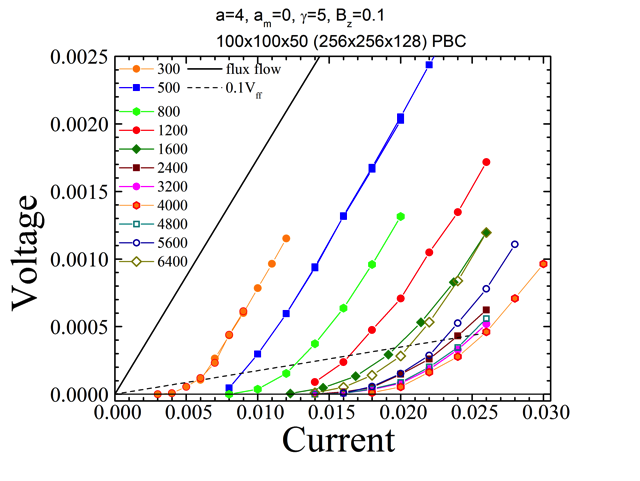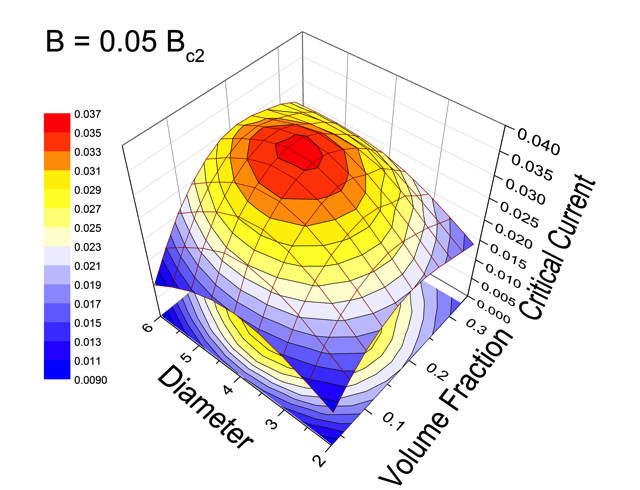Optimizing superconductor transport properties through large-scale simulation

# Optimization of pinning by spherical nanoparticles

## Motivation

Pinning by self-assembled nanoparticles has emerged as one of most efficient routes to enhance current-carrying capability of practical superconductors. At present, improvement is achieved mostly by trial-and-error approach without deep understanding of underlying pinning mechanisms. Theoretical estimates are available only in simple limiting cases such as small concentration of the particles.

## Approach and goal

Using the TDGL model, we systematically explore dependence of the critical current on size and concentration of particles in order to find optimal pinning configuration for every magnetic field.

This animation illustrates motion of vortex lines near the critical current through the superconducting sample containing 1600 nanoparticles with diameter a = 4ξ for the magnetic field B = 0.1Hc2. The system size is 100×100×50 ξ3 (256×256×128 mesh points).Series of the voltage-current dependences for different number of particles N used for evaluation of the critical current. One can see that there is an optimal concentration of particles for best pinning, corresponding to N = 4000.Surface plot of the critical current for fixed magnetic field on the diameter of particles and volume fraction occupied by them. One can see that maximum critical current is achieved when particles with diameter a = 4.5ξ occupy 20% of the volume.

This animation illustrates evolution of vortex dynamics near the critical currents for two cases: (i) increasing magnetic field for fixed number of spherical particles, N = 500 and (ii) increasing number of particles for fixed magnetic field B = 0.016Hc2. In both cases the particle diameter is 4ξ and the system size is 100×100×50 ξ3 (256×256×128 mesh points).